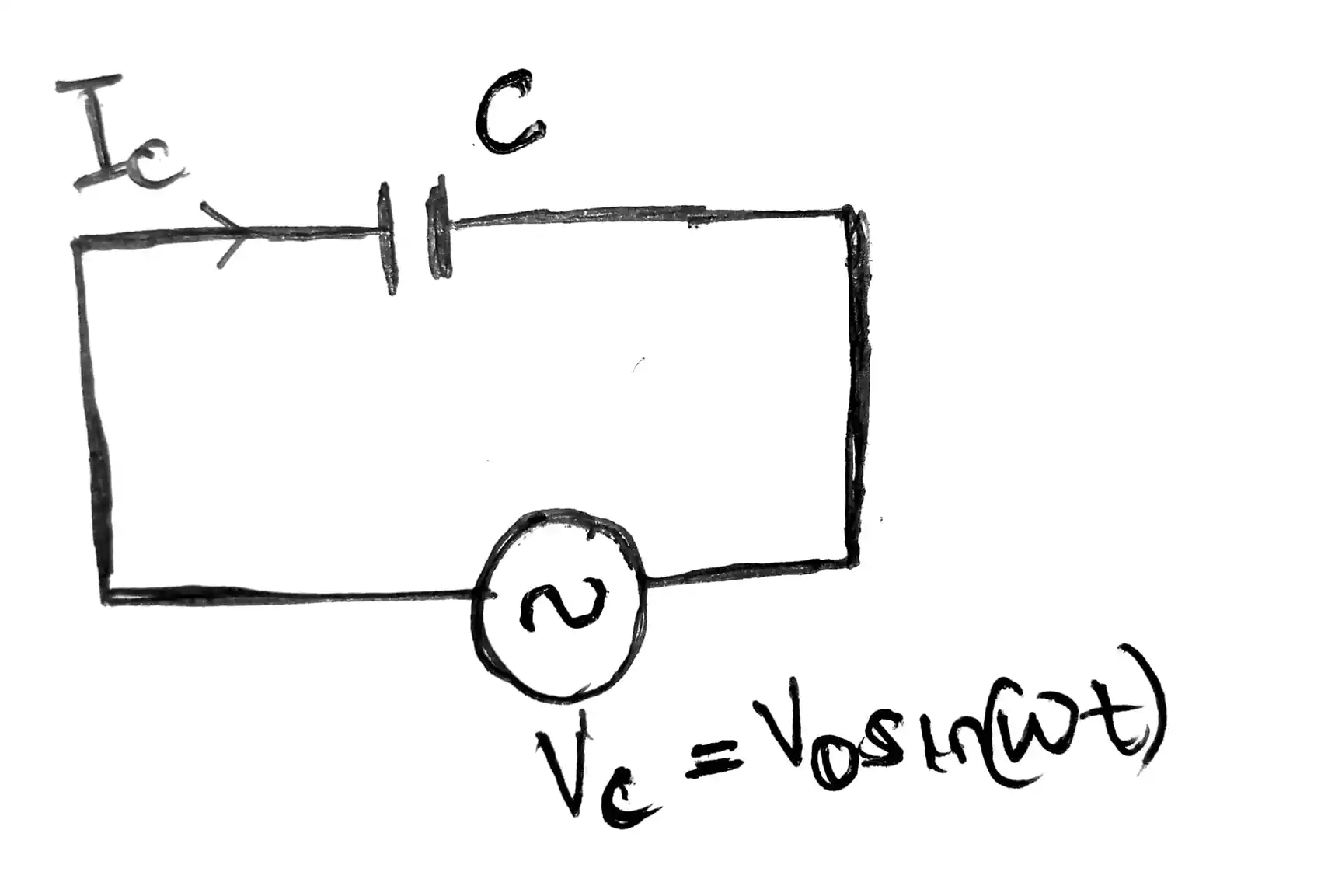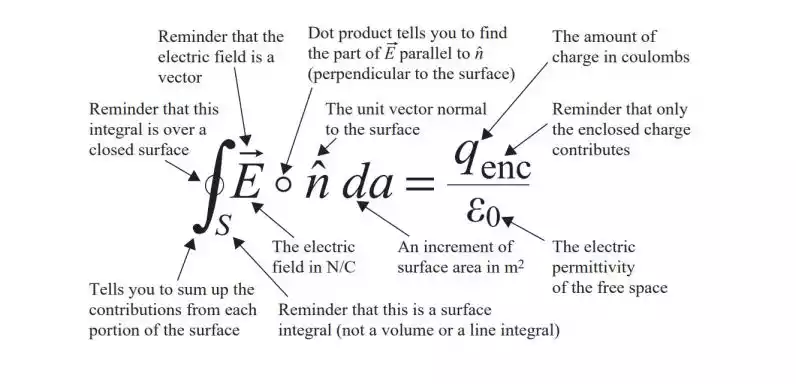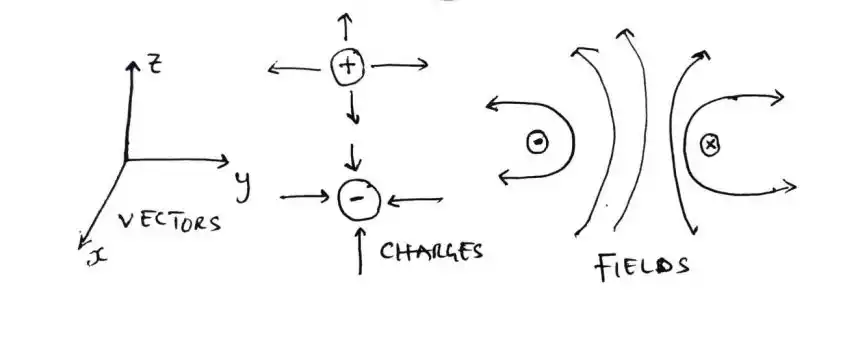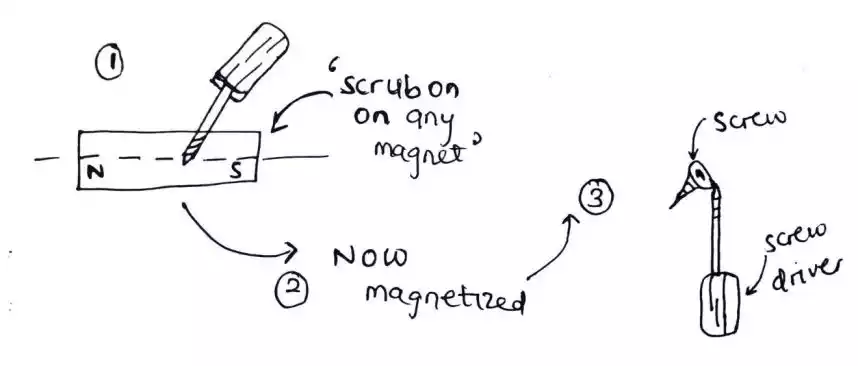# Solved: Verify that the conduction current in the wire equals the displacement current ...

## Question:

Verify that the conduction current in the wire equals the displacement current between the plates of the parallel plate capacitor in the figure below.

The voltage source supplies $V_c = V_o\sin\omega {t}$.## Solution:

The question is asking us to compare if the conduction current $I_c$ and the displacement current $I_d$ are equal.

Thus, we need to calculate for each.

### 1. Conduction current (Ic) = ?

From the circuit, we can see that, the current flowing in the circuit is the same as the current passing through the capacitor.

Hence, we can use $I_c = C\frac{\text{d}V_c}{\text{d}t}$.

Where:

$C$ is capacitance of the capacitor. $C =\frac{ \epsilon A}{d}$

$V_c$ is the voltage across the capacitor, which is the same as the input voltage source $V_c = V_o\sin\omega{t}$.

Therefore, $I_c = C\frac{d(V_o\sin\omega t)}{dt}$

If we differentiate the voltage, we have $I_c = C V_o \omega\cos\omega t$.

Now let’s find the displacement current $I_d$.

### 2. Displacement current $(I_d)$ = ?

But $I_d = \int\int J_d \cdot ds$ (surface integral of displacement current density over a surface)

This is also equal to the surface integral of $\int\int\frac{\partial D}{\partial t} \cdot ds$

Where:

$D$ (is electric flux density). It can also be expressed in terms of electric field intensity ($E$) as $D = \epsilon E$

Hence, $I_d = \int\int J_d \cdot ds = \int\int\frac{\partial D}{\partial t} \cdot ds$.

But $E$ can also be expressed in terms of $\frac{V }{D} \frac{(voltage [V])}{(distance [m])}$

Hence, $D = \epsilon\frac{V }{D} \frac{(voltage [V])}{(distance [m])}$

So to solve for $I_d = \int\int\frac{\partial D}{\partial t} \cdot ds$, we need to differentiate $D$.

Therefore, $I_d = \int\int\frac{\partial D}{\partial t} \cdot ds$.

$\frac{\partial D}{\partial t} = \frac{\epsilon\cdot V_o}{d} \omega\cos\omega t$

$I_d = \int\int(\frac{\epsilon\cdot V_o}{d} \omega\cos\omega t) \cdot ds$

$= \int\int ds (\frac{\epsilon\cdot V_o}{d} \omega\cos\omega t)$.

$\int\int ds$ = Area of the surface $(A)$

By substituting $A$, $I_d = A (\frac{\epsilon\cdot V_o}{d} \omega\cos\omega t)$

Remember that $C = \frac{\epsilon A}{d}$

Therefore, $I_d = C V_o\omega\cos\omega t$.

So in conclusion, by comparing $I_c$ and $I_d$, we can see that they are equal. i.e., $I_c = I_d = C V_o\omega\cos\omega t$.What is the Importance of Maxwell's Equations in Electromagnetism?Resources to help you learn and understand Electromagnetics field theory from scratchThe Easiest Way to Study Electromagnetics Theory10 Important facts you need to know about magnets and magnetic fields